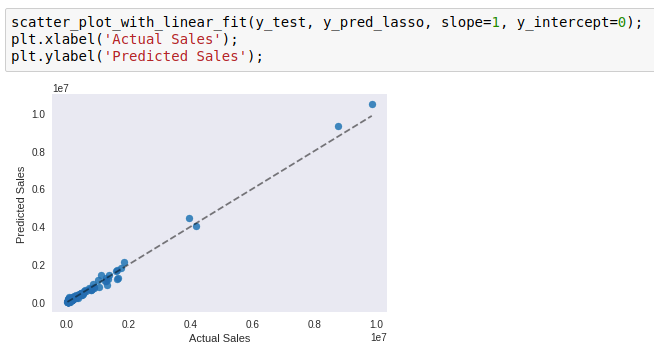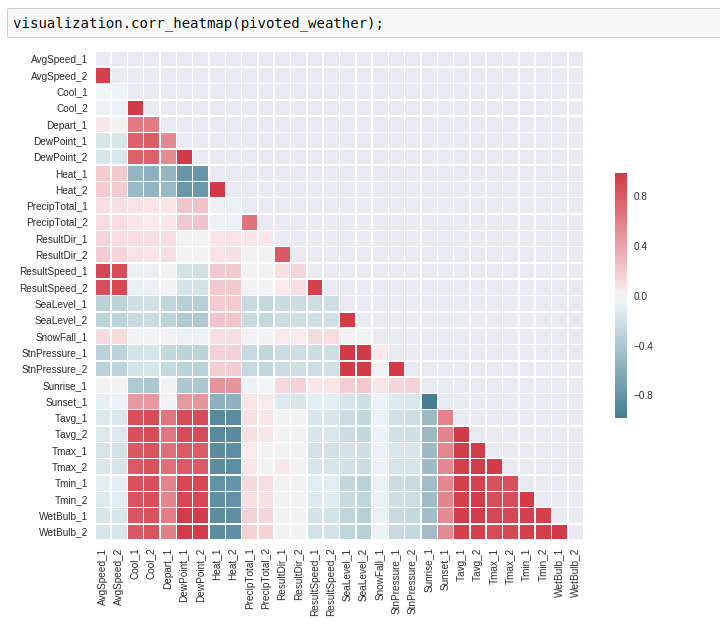In this blog I’ll share a few plotting functions I regularly use.

### Scatter Plot with Linear Fit

I originally wrote this function to plot scatter plots of two variables plus a linearly fitted line. But later I realized `seaborn` already has `regplot` and `lmplot` that do the same thing but better. You can read more about them in this tutorial (and learn not to re-invent the wheel!). I have repurposed it to visualize model evaluation. When your `y` is a continuous variable, you can plot the predicted `y` values on one axis, and the actual `y` values on another, and see how well they line up along `y=x`. To plot the `y=x` line, simply set `slope=1`, `y_intercept=0`.

Here is an example:### Correlation Heatmap

This is a wrapper function for `seaborn`’s `heatmap`. I use it to look at the correlations between variables in a pandas DataFrame, which is quite useful during exploratory data analysis.

Here is an example:When you see highly correlated variables, you have to be very careful if you are using a linear regression model (the LINE assumptions!). Plotting a heatmap is a quick way to check for that.